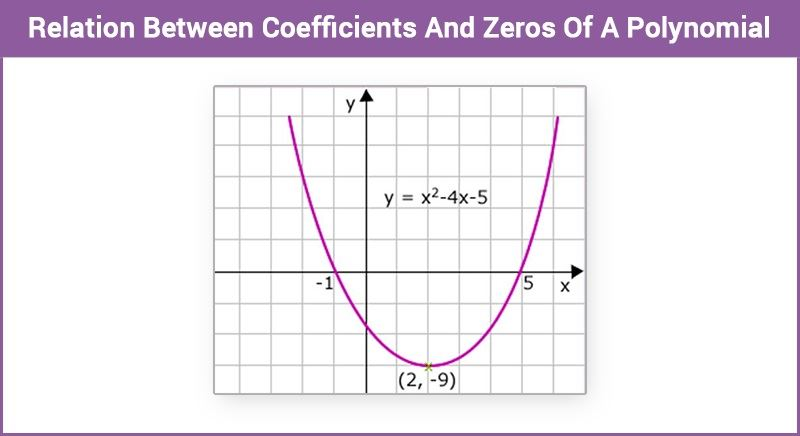# Relation Between Coefficients And Zeros Of A Polynomial

A linear polynomial of the form P(x) = ax + b. If k is the zero of P(x), then,

P(k) = ak + b = 0

Zero of the polynomial, k = – b/a = – constant term/coefficient ofx

Now, consider the quadratic polynomial, P(x) = $4x^2-9x+2$

Factorisation of P(x) can be done by splitting the middle term into two terms such that their product is a multiple of the first term. ie. multiple of $4x^2$. Middle term -9x can be written as,

$-9x$ = $-8x-x[-8x×-x=8x^2=2×4x^2]$

$4x^2-9x+2$ = $4x^2-8x-x+2$

= $4x (x-2)-(x-2)$

= $(4x-1)(x-2)$

Zeros of the polynomial $4x^2-9x+2$ will be same as zeros of $(4x-1)(x-2)$.

Zeros are found by equating the polynomial to zero.i.e.

$(4x-1)(x-2)$ = 0

Therefore, either $(4x-1)$ = 0 or $(x-2)$ = 0

4x – 1 = 0 gives x = $\frac 14$ and x – 2 = 0 gives x = 2.

Zeros of P(x) are $\frac 14$ and 2.

It is observed that, sum of zeros, $\frac 14 +2$ = $\frac 94$ = –$\frac {coefficient of x}{coefficient of x^2 }$

Product of the roots,1/4×2=1/2 = constant term/coefficient of x^2

Let’s take one more example to verify above concept, let P(x) = $x^2-3x-10$.

To factorise the above polynomial, we have to split the middle term -3x into two terms such that the product of them is a multiple of $-10x^2$.

Therefore,

-3x can be written as -3x =-5x + 2x, [since -5x × 2x = $-10x^2]$

$x^2-3x-10$ =$x^2-5x+2x-10$

= x (x – 5) + 2(x – 5)

= (x – 5)(x + 2)

Zeros of P(x) are,

x – 5 = 0, x = 5

x + 2 = 0, x=-2

Sum of zeros,

5 – 2 = $\frac 31$ = $-\frac {coefficient of x}{coefficient of x^2 }$

Product of zeros,

5 × -2 = – $\frac {10}{1}$= constant term/coefficient of x^2

In general, if α and β are the zeros of the polynomial P(x)= $ax^2+bx+c,a≠0$, then (x – α) and (x – β) are the factors of P(x).

P(x) can be written as,

$ax^2+bx+c=k(x-α)(x-β)$, where k is a constant.

= $k[x^2-(α+β)x+αβ]$

=$kx^2-(α+β)kx+kαβ$

Comparing the coefficients of terms gives,

a = k, b = -k(α + β), c = kαβ

It gives,

α + β= – $\frac bk$ =- $\frac ba$

αβ = $\frac ck$ = $\frac ca$

Therefore,

Sum of zeros,

α + β = – b/a = $- \frac{coefficient\; of\; x}{coefficient \;of\; x^2}$

Product of zeros,

αβ = c/a =$\frac {constant\; term}{coefficient\; of\; x^2 }$

## Solved Examples:

Example 1: Find a quadratic polynomial whose sum and product of zeros are 7 and 12.

Let α and β be zeros of polynomial of form $ax^2+bx+c$,

α + β = 7 = $- \frac ba$

α + β = 12 = $\frac ca$

If a = 1, then b = -7 and c = 12

Therefore, one quadratic polynomial satisfying the above condition is $x^2-7x+12$

Now, consider the cubic polynomial P(x) = $ax^3+bx^2+cx+d$,a ≠ 0. If α,β,and γare zeros of P(x), then,

α + β + γ = $– \frac ba$

αβ + βγ + αγ = $\frac ca$

αβγ = $– \frac da$

Example 2 : Two zeros of the polynomial P(x) = $x^3-4x^2+x+6$ are 2 and -1. Find the third zero of P(x).

Let the third root be γ,

Comparing polynomial with $ax^3+bx^2+cx+d$ gives,

a = 1, b = -4, c = 1, d = 6

Sum of zeros of the cubic polynomial

2 – 1 + γ = $- \frac ba$ = 4

γ = 4 – 1 = 3

To know more about quadratic and cubic polynomial, log onto www.byjus.com and learn things in a better way.